Related Articles

# PHP – Mysql Joins

• Last Updated : 31 Mar, 2021

In this article, we are going to join two tables using PHP and display them on the web page.

Introduction :

PHP is a server-side scripting language, which is used to connect with databases. Using this, we can get data from the database using PHP scripts. The database language that can be used to communicate with PHP is MySQL. MySQL is a database query language that is used to manage databases.

Requirements :

Xampp server – xampp server is used to store our database locally.  We are going to access the data from xampp server using PHP.

In this article, we are taking the student details database that contains two tables. They are student_address and student_marks.

Structure of tables :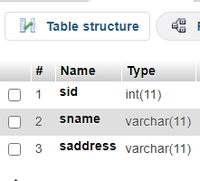table2=student_marks.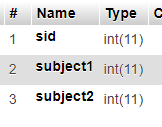We are going to perform INNER JOIN, LEFT JOIN, RIGHT JOIN on these two tables.

1. INNER JOIN :

The INNER JOIN is a keyword that selects records that have matching values in both tables.

Syntax :

```SELECT column 1,column 2,...column n
FROM table1
INNER JOIN table2
ON table1.column_name = table2.column_name;```

Example :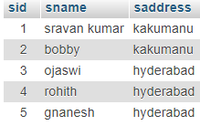And student_marks table includes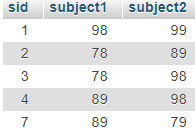By using sid, we can join these two tables using an Inner join, since, sid is common in two tables.

• Query to display student_address details based on inner join –
`SELECT  * from student_address INNER JOIN student_marks on student_address.sid=student_marks.sid;`

Result :

```STUDENT-ID : 1 ----- NAME : sravan kumar ----- ADDRESS : kakumanu
STUDENT-ID : 2 ----- NAME : bobby ----- ADDRESS : kakumanu
• Query to display student_marks details based on inner join.
`SELECT  * from student_marks INNER JOIN student_address on student_address.sid=student_marks.sid`

Result :

```STUDENT-ID : 1 ----- SUBJECT 1 : 98 ----- SUBJECT 2 : 99
STUDENT-ID : 2 ----- SUBJECT 1 : 78 ----- SUBJECT 2 : 89
STUDENT-ID : 3 ----- SUBJECT 1 : 78 ----- SUBJECT 2 : 98
STUDENT-ID : 4 ----- SUBJECT 1 : 89 ----- SUBJECT 2 : 98```

2. LEFT JOIN :

The LEFT JOIN keyword is used to return all records from the left table (table1), and the matching records from the right table (table2).

Syntax :

```SELECT column1,column2,...columnn
FROM table1
LEFT JOIN table2
ON table1.column_name = table2.column_name;```
• Query to display all student_address table based on student id using  left join
`SELECT * from student_address LEFT JOIN student_marks on student_address.sid=student_marks.sid`

Result :

```STUDENT-ID : 1 ----- NAME : sravan kumar ----- ADDRESS : kakumanu
STUDENT-ID : 2 ----- NAME : bobby ----- ADDRESS : kakumanu
• Query to display all student_marks  table based on student id using left join
`SELECT * from student_marks LEFT JOIN student_address on student_address.sid=student_marks.sid`

Result :

```STUDENT-ID : 1 ----- SUBJECT 1 : 98 ----- SUBJECT 2 : 99
STUDENT-ID : 2 ----- SUBJECT 1 : 78 ----- SUBJECT 2 : 89
STUDENT-ID : 3 ----- SUBJECT 1 : 78 ----- SUBJECT 2 : 98
STUDENT-ID : 4 ----- SUBJECT 1 : 89 ----- SUBJECT 2 : 98
STUDENT-ID : ----- SUBJECT 1 : 89 ----- SUBJECT 2 : 79```

3. RIGHT JOIN :

The RIGHT JOIN keyword is used to return all records from the right table (table2), and the matching records from the left table (table1).

Syntax :

```SELECT column1,column2,...columnn
FROM table1
RIGHT  JOIN table2
ON table1.column_name = table2.column_name;```
• Query to display all student_address table based on student id using  right join
`SELECT * from student_address RIGHT JOIN student_marks on student_address.sid=student_marks.sid`

Result :

```STUDENT-ID : 1 ----- NAME : sravan kumar ----- ADDRESS : kakumanu
STUDENT-ID : 2 ----- NAME : bobby ----- ADDRESS : kakumanu
STUDENT-ID : 7 ----- NAME : ----- ADDRESS :```
• Query to display all student_marks  table based on student id using right join
`SELECT * from student_marks RIGHT JOIN student_address on student_address.sid=student_marks.sid`

Result :

```STUDENT-ID : 1 ----- SUBJECT 1 : 98 ----- SUBJECT 2 : 99
STUDENT-ID : 2 ----- SUBJECT 1 : 78 ----- SUBJECT 2 : 89
STUDENT-ID : 3 ----- SUBJECT 1 : 78 ----- SUBJECT 2 : 98
STUDENT-ID : 4 ----- SUBJECT 1 : 89 ----- SUBJECT 2 : 98
STUDENT-ID : 5 ----- SUBJECT 1 : ----- SUBJECT 2 :```

Approach :

• Create a database named database and create tables(student_address and student_marks)
• Insert records into two tables using PHP
• Write SQL  query to perform all joins using PHP
• Observe the results.

Steps:

• Start xampp server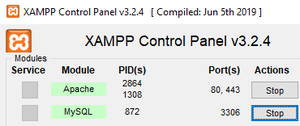• Type “localhost/phpmyadmin” in your browser and create a database named “database” then create two tables named student_address and student_marks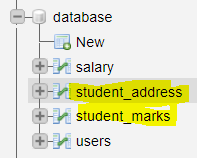Student_marks table structure :• Insert the records into the student_address table using PHP (data1.php) Run code by typing “localhost/data1.php”

## PHP

 `connect_error) {``  ``die``(``"Connection failed: "` `. ``\$conn``->connect_error);``}``//insert records into table``\$sql`  `= ``"INSERT INTO student_address VALUES (1,'sravan kumar','kakumanu');"``;``\$sql` `.= ``"INSERT INTO student_address VALUES (2,'bobby','kakumanu');"``;``\$sql` `.= ``"INSERT INTO student_address  VALUES (3,'ojaswi','hyderabad');"``;``\$sql` `.= ``"INSERT INTO student_address  VALUES (4,'rohith','hyderabad');"``;``\$sql` `.= ``"INSERT INTO student_address  VALUES (5,'gnanesh','hyderabad');"``;`` ` `if` `(``\$conn``->multi_query(``\$sql``) === TRUE) {``  ``echo` `"data stored successfully"``;``} ``else` `{``  ``echo` `"Error: "` `. ``\$sql` `. ``"
"` `. ``\$conn``->error;``}`` ` `\$conn``->close();``?>`

Output :Write PHP code to insert details in the student_marks table. (data2.PHP)

## PHP

 `connect_error) {``  ``die``(``"Connection failed: "` `. ``\$conn``->connect_error);``}``//insert records into table``\$sql`  `= ``"INSERT INTO student_marks VALUES (1,98,99);"``;``\$sql` `.= ``"INSERT INTO student_marks VALUES (2,78,89);"``;``\$sql` `.= ``"INSERT INTO student_marks  VALUES (3,78,98);"``;``\$sql` `.= ``"INSERT INTO student_marks  VALUES (4,89,98);"``;``\$sql` `.= ``"INSERT INTO student_marks  VALUES (7,89,79);"``;`` ` `if` `(``\$conn``->multi_query(``\$sql``) === TRUE) {``  ``echo` `"data stored successfully"``;``} ``else` `{``  ``echo` `"Error: "` `. ``\$sql` `. ``"
"` `. ``\$conn``->error;``}`` ` `\$conn``->close();``?>`

Output :

Type “localhost/data2.php” to see the output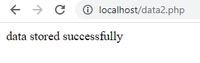Write PHP code to perform inner join (form.php)

## PHP

 `````"``;``echo` `"
"``;``//sql query to display all student_address table based on student id using  inner join``\$sql` `= ``"SELECT  * from student_address INNER JOIN student_marks on student_address.sid=student_marks.sid"``;``\$result` `= ``\$conn``->query(``\$sql``);``//display data on web page``while``(``\$row` `= mysqli_fetch_array(``\$result``)){``    ``echo` `" STUDENT-ID : "``. ``\$row``[``'sid'``], ``" ----- NAME : "``. ``\$row``[``'sname'``] ,``" ----- ADDRESS : "``. ``\$row``[``'saddress'``] ;``    ``echo` `"
"``;``     ` `}`` ` `echo` `"
"``;``echo` `"inner join on student_marks: "``;``echo` `"
"``;``echo` `"
"``;``//sql query to display all student_marks  table based on student id using  inner join``\$sql1` `= ``"SELECT  * from student_marks INNER JOIN student_address on student_address.sid=student_marks.sid"``;``\$result1` `= ``\$conn``->query(``\$sql1``);``//display data on web page``while``(``\$row` `= mysqli_fetch_array(``\$result1``)){``    ``echo` `" STUDENT-ID : "``. ``\$row``[``'sid'``], ``" ----- SUBJECT 1 : "``. ``\$row``[``'subject1'``] ,``" ----- SUBJECT 2 : "``. ``\$row``[``'subject2'``] ;``    ``echo` `"
"``;``     ` `}`` ` ` ` `//close the connection`` ` `\$conn``->close();``?>`````

Output :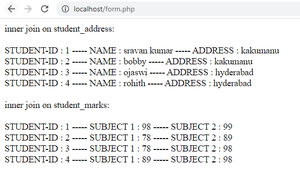Write code to perform right join (form1.php)

## PHP

 `````"``;``echo` `"
"``;``//sql query to display all student_address table based on student id using  right join``\$sql` `= ``"SELECT  * from student_address RIGHT JOIN student_marks on student_address.sid=student_marks.sid"``;``\$result` `= ``\$conn``->query(``\$sql``);``//display data on web page``while``(``\$row` `= mysqli_fetch_array(``\$result``)){``    ``echo` `" STUDENT-ID : "``. ``\$row``[``'sid'``], ``" ----- NAME : "``. ``\$row``[``'sname'``] ,``" ----- ADDRESS : "``. ``\$row``[``'saddress'``] ;``    ``echo` `"
"``;``     ` `}`` ` `echo` `"
"``;``echo` `"right  join on student_marks: "``;``echo` `"
"``;``echo` `"
"``;``//sql query to display all student_marks  table based on student id using right join``\$sql1` `= ``"SELECT  * from student_marks RIGHT JOIN student_address on student_address.sid=student_marks.sid"``;``\$result1` `= ``\$conn``->query(``\$sql1``);``//display data on web page``while``(``\$row` `= mysqli_fetch_array(``\$result1``)){``    ``echo` `" STUDENT-ID : "``. ``\$row``[``'sid'``], ``" ----- SUBJECT 1 : "``. ``\$row``[``'subject1'``] ,``" ----- SUBJECT 2 : "``. ``\$row``[``'subject2'``] ;``    ``echo` `"
"``;``     ` `}`` ` ` ` `//close the connection`` ` `\$conn``->close();``?>`````

Output :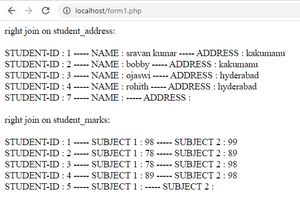Write PHP code to perform left join (form2.php)

## PHP

 `````"``;``echo` `"
"``;``//sql query to display all student_address table based on student id using  left join``\$sql` `= ``"SELECT  * from student_address LEFT JOIN student_marks on student_address.sid=student_marks.sid"``;``\$result` `= ``\$conn``->query(``\$sql``);``//display data on web page``while``(``\$row` `= mysqli_fetch_array(``\$result``)){``    ``echo` `" STUDENT-ID : "``. ``\$row``[``'sid'``], ``" ----- NAME : "``. ``\$row``[``'sname'``] ,``" ----- ADDRESS : "``. ``\$row``[``'saddress'``] ;``    ``echo` `"
"``;``     ` `}`` ` `echo` `"
"``;``echo` `"left join on student_marks: "``;``echo` `"
"``;``echo` `"
"``;``//sql query to display all student_marks  table based on student id using left join``\$sql1` `= ``"SELECT  * from student_marks LEFT JOIN student_address on student_address.sid=student_marks.sid"``;``\$result1` `= ``\$conn``->query(``\$sql1``);``//display data on web page``while``(``\$row` `= mysqli_fetch_array(``\$result1``)){``    ``echo` `" STUDENT-ID : "``. ``\$row``[``'sid'``], ``" ----- SUBJECT 1 : "``. ``\$row``[``'subject1'``] ,``" ----- SUBJECT 2 : "``. ``\$row``[``'subject2'``] ;``    ``echo` `"
"``;``     ` `}`` ` ` ` `//close the connection`` ` `\$conn``->close();``?>`````

Output :

type  localhost/form2.php in browser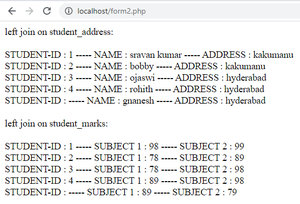My Personal Notes arrow_drop_up